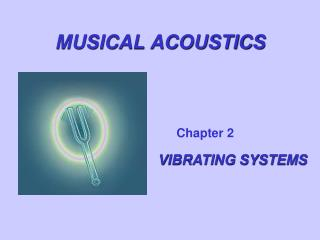Download PresentationMUSICAL ACOUSTICS

# MUSICAL ACOUSTICS - PowerPoint PPT PresentationDownload Presentation## MUSICAL ACOUSTICS

- - - - - - - - - - - - - - - - - - - - - - - - - - - E N D - - - - - - - - - - - - - - - - - - - - - - - - - - -
##### Presentation Transcript

1. MUSICAL ACOUSTICS Chapter 2 VIBRATING SYSTEMS

2. SIMPLE HARMONIC MOTION A simple vibrator consisting of a mass and a spring. At equilibrium (center), the upward force exerted by the spring and the force of gravity balance each other, and the net force F on the mass is zero.

3. Simple Harmonic Motion Graphs of simple harmonic motion: (a) Displacement versus time (b) Speed versus Time. Note that speed reaches its maximum when displacement is zero and vice versa.

4. Vibratory motion: y,v, and a all change with time.

5. EVERY VIBRATING SYSTEM HAS Inertia (mass) Elasticity (spring) Hooke’s Law F =Ky For a mass/spring In Chapter 1 we learned that KE= ½ mv2 Similarly, it can be shown that PE = ½ Ky2 If the vibrator has damping:

6. A mass hangs from a spring. You raise the mass 1 cm, hold it there for a short time and then let it drop Make a graph of its motion Make a graph of its total energy.

7. SIMPLE VIBRATING SYSTEMS A simple pendulum

8. A mass-spring system vibrates at a frequencyf • If the mass is doubled: • The frequency will be 2f • The frequency will be √2f • The frequency will remain f • The frequency will be f/√2 • e) The frequency will be f/2 A mass swings on the end of a string at frequency f • If the mass is doubled: • The frequency will be 2f • b) The frequency will be √2f • c)The frequency will remain f • The frequency will be f/√2 • e) The frequency will be f/2

9. SIMPLE VIBRATING SYSTEMS A piston free to vibrate in a cylinder

10. SIMPLE VIBRATING SYSTEMS A piston free to vibrate in a cylinder A Helmholtz resonator

11. SIMPLE VIBRATING SYSTEMS A piston free to vibrate in a cylinder A Helmholtz resonator m=ρɑl K=ρɑ2l 2/V

12. SYSTEMS WITH TWO MASSES

13. Longitudinal vibrations of a three-mass vibrator Transverse vibration of a three-mass vibratorTransverse vibrations for spring systems with multiple masses

14. LINEAR ARRAY OF OSCILLATORS

15. MODES OF CIRCULAR MEMBRANES

16. SNARE DRUM TIMPANI BASS DRUM

17. VIBRATING BARS Both ends free One end clamped Arrows locate the nodes

18. CHLADNI PATTERNS OF A CIRCULAR PLATE SALT COLLECTS AT THE NODES